# GMAT Math : Understanding rays

## Example Questions

2 Next →

### Example Question #11 : Understanding Rays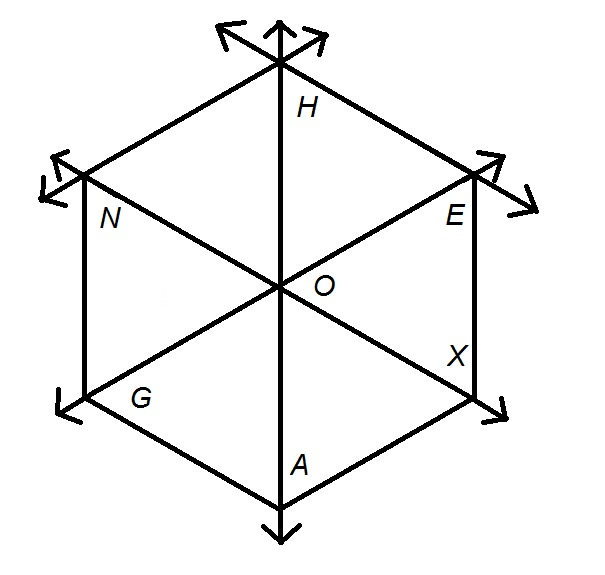In the above figure, give the union of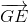and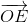.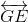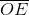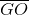Explanation:can be seen to be completely contained in- that is,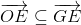. The union of a set and its subset is the containing set, so the correct response is.

### Example Question #12 : Understanding RaysGive the union of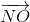and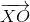in the above figure.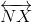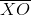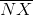Explanation:

The diagram below showsandin red and green, respectively: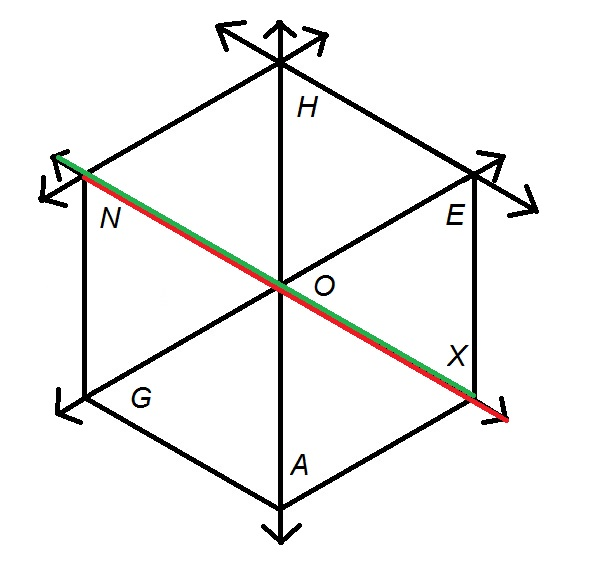The union of the two sets is the set of points in one or the other; this set is the entire line containing the two rays, which is.

### Example Question #13 : Understanding RaysIn the above figure, letbe the midpoint of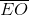. Which of the following would give another name for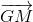?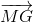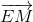Explanation:

Below is the diagram with midpointofadded; also,, the ray starting at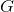and passing through, is in green.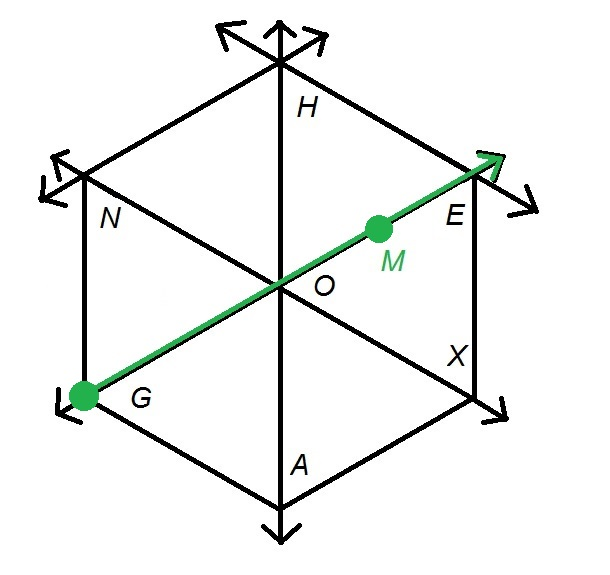A ray is named after, in order, its endpoint and any other point on the ray.hasas an endpoint, and also includes the points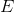and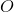, so there are two valid alternative names,and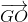, among the choices. The correct response is.

### Example Question #14 : Understanding RaysIn the above diagram, let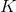andbe the midpoints of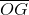and, respectively, andand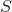be the midpoints of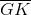and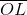, respectively. Which of the following is not a valid alternative name for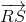?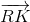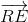Each of the other choices gives a valid alternative name for.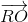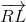Each of the other choices gives a valid alternative name for.

Explanation:

Below is the diagram with the points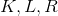, and, as described, shown in green. Also,, the ray that has endpointand passes through, is marked in red.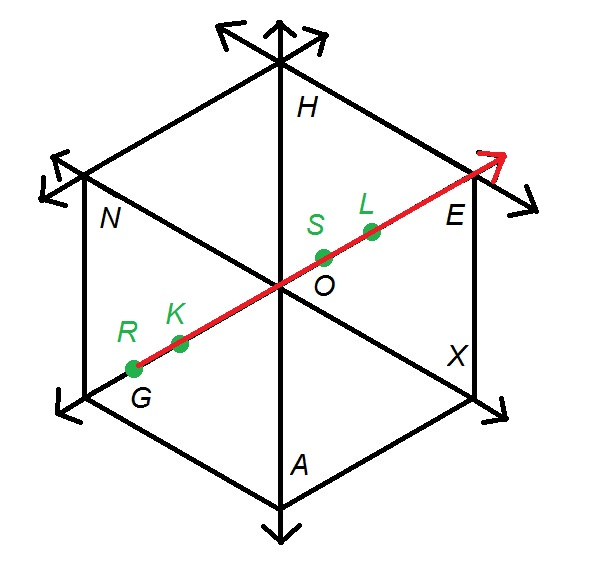The ray also passes through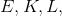and, so, and—all four given names—are also valid names for the ray.

2 Next →

Tired of practice problems?

Try live online GMAT prep today.